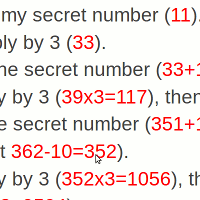# "Threes" Birthday Math Trick

### Seem like a math genius in front of your friends!

By

Here's a math trick that is easy to remember, and will bedazzle your friends with your pure math genius - if you're a kid! It only works for kids, so sorry mom and dad! Your friends will know you got it from the 'net, so give it to your son or daughter, and let them soak up the glory!

It's not the only similar math trick on this site - check out this one too!

So, if you're ready, study the steps below, practice with mom or dad, or a close friend, until you're sure you can get it right every time!

• First, ask your friend to choose a number from 1 to 20 (no decimal points allowed!), and write it on a piece of paper. Make sure they hide the paper from you! Tell your friend that you, the mathemagician, are going to use your pure math genius to figure it out!
• Enter the secret number
• Multiply by 3
• Add the secret number, then subtract 5.
• Multiply by 3, then multiply by 3 again.
• Add the secret number, then subtract the number of their favourite month (you don't have to know what month that is!)
• Multiply by 3, then multiply by 3 again, and then again a third time.
• Add the secret number, then subtract their favourite day of the month (again, you don't have to know what it is).
• Ask them to show you the result.
• Now, you can guess their secret number... The number on the calculator will be quite big, but that's no sweat - it will just make you seem like more of a genius!
• Here's how you figure it out :
• If the result is negative, their secret number was 1 (or they cheated)
• If the result has only three digits, their secret number was 2
• Otherwise, you ignore the last three digits, and then add 2 to get their secret number!
Here's a couple of examples, to show you how it works!
• You're playing the trick on me, and I choose the secret number 11.
• Then, you give me the instructions. I'll write the calculator's answers in red.
• I enter my secret number (11).
• I multiply by 3 (33).
• I Add the secret number (33+11=44), then subtract 5 (44-5=39).
• Multiply by 3 (39x3=117), then multiply by 3 again (117x3=351).
• Add the secret number (351+11=362), then subtract the number of their favourite month (I'll choose October, and get 362-10=352).
• Multiply by 3 (352x3=1056), then multiply by 3 again (1056x3=3168), and then again a third time (3168x3=9504).
• Add the secret number (9504+11=9515), then subtract their favourite day of the month (I'll choose the 23rd, so 9515-23=9492).
• Now, I show you the result. In your head (this is easy), you throw away the last three digits (9492), and add 2 (9+2=11 Tadaaah!)
• You put on your best mathemagical frown of genius concentration, the announce the answer! I stand amazed! I bow to your superior intellect! (Just don't tell me the last two steps, or you'll give it all away :-)

If the secret number had been 2, and I still chose October 23, the answer would have been 492. If you toss out the last three digits, there's nothing left - the secret number is therefore 0+2=2

If the secret number is 1, you'll actually have a negative answer on the calculator at the end, say -508. Try it! That's how you'll know the secret number is 1.

Actually, this trick can handle numbers much bigger than 20. But if the calculator shows 354920492, and you say the answer is 354922, it would give the secret away, don't you think?

If you're interested, here's a variation of this trick, that lets you extract people's birthdays. The mental math at the end is a little trickier, but you'll still be able to manage it, I'm sure!

Your friend should enter the following steps (I show in red what the calculator should show, if your friend's birthday is February 29).

• Enter the month you were born (2)
• Multiply by 3 (2x3=6)
• Add the month again (6+2=8), then subtract 4 (8-4=4).
• Multiply by 3 (4x3=12), then again by 3 (12x3=36).
• Add the month again (36+2=38), then subtract 1 (38-1=37).
• Multiply by 3 again (37x3=111)
• Now, add the day of their birthday (I'm using February 29 as an example, so 111+29=140).
• Multiply by 3 again (140x3=420), and again (420x3=1260).
• Finally, subtract 12 (1260-12=1248), then add the month and the day they were born (1248+2=1250, then 1250+29=1279).
If your friend followed these steps correctly, then you can do some simple sums in your head to figure out their birthday :-
• Add one (1279+1=1280). This should always give a number with a 0 on the end.
• Divide by 10 (1280 / 10 = 128), then finally,
Put a slash between the tens and hundreds digits, and you have your friend's birthday, 2/29!

Of course, if you are in Europe or Asia or Australia, you'll want the 29th February to look like 29/2. To get that, just swap the words month and day in the above instructions, like so.

• Enter the monthday you were born (29)
• Multiply by 3 (29x3=87)
• Add the monthday again (87+29=116), then subtract 4 (116-4=112).
• Multiply by 3 (112x3=336), then again by 3 (336x3=1008).
• Add the monthday again (1008+29=1037), then subtract 1 (1037-1=1036).
• Multiply by 3 again (1036x3=3108)
• Now, add the daymonth of their birthday (3108+2=3110).
• Multiply by 3 again (3110x3=9330), and again (9330x3=27990).
• Finally, subtract 12 (27990-12=27978), then add the day and the month they were born (27978+29=28007, then 28007+2=28009).
• Divide by 10 (28010 / 10 = 2801), then finally,